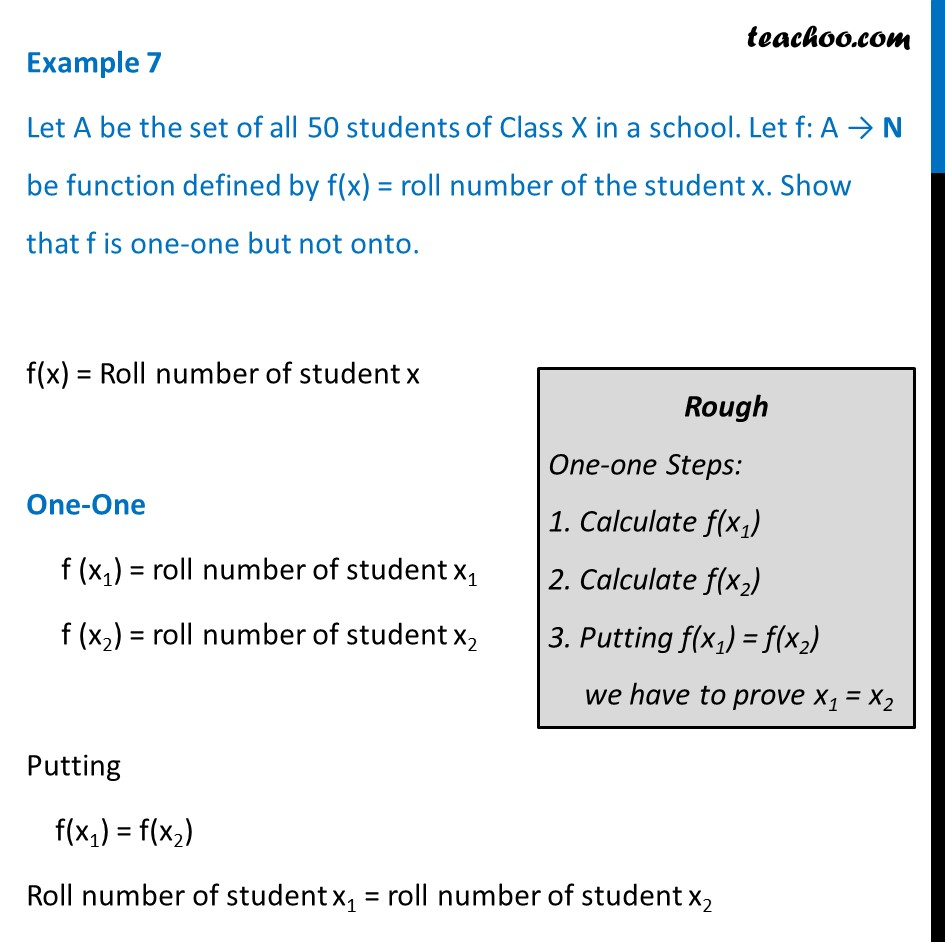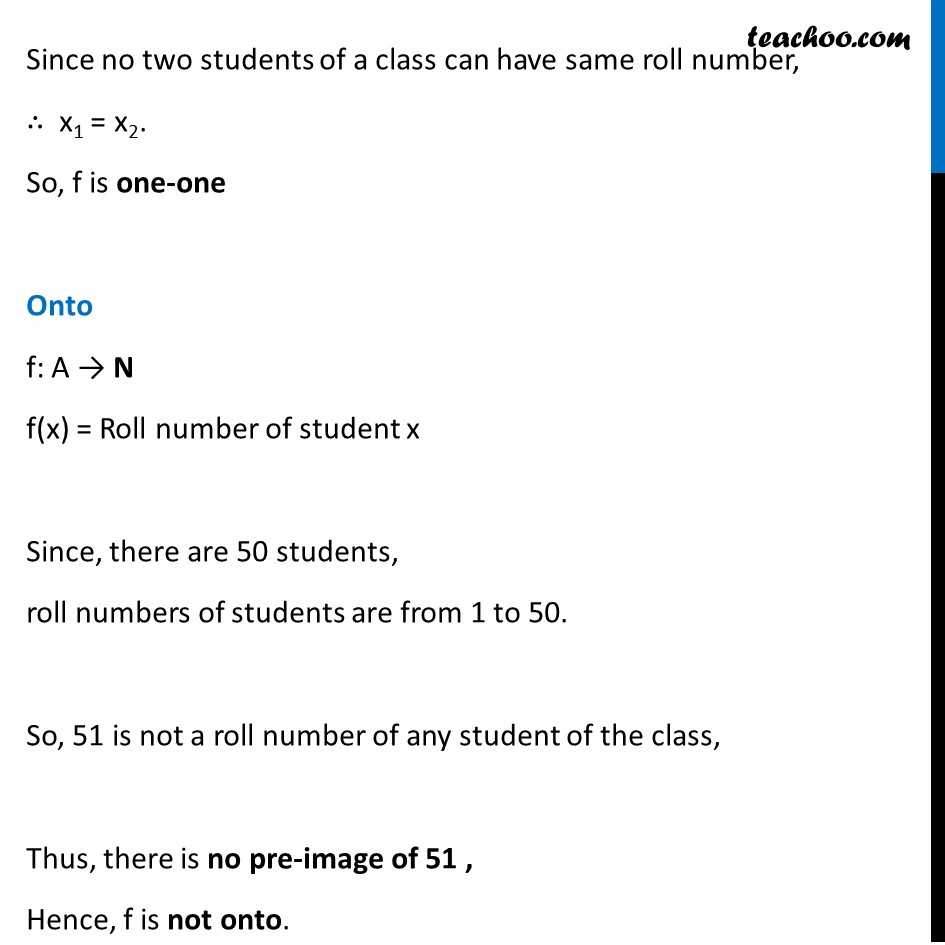To prove one-one & onto (injective, surjective, bijective)

Chapter 1 Class 12 Relation and Functions
Concept wiseLearn in your speed, with individual attention - Teachoo Maths 1-on-1 Class

### Transcript

Example 7 Let A be the set of all 50 students of Class X in a school. Let f: A → N be function defined by f(x) = roll number of the student x. Show that f is one-one but not onto. f(x) = Roll number of student x One-One f (x1) = roll number of student x1 f (x2) = roll number of student x2 Putting f(x1) = f(x2) Roll number of student x1 = roll number of student x2 Since no two students of a class can have same roll number, ∴ x1 = x2. So, f is one-one Onto f: A → N f(x) = Roll number of student x Since, there are 50 students, roll numbers of students are from 1 to 50. So, 51 is not a roll number of any student of the class, Thus, there is no pre-image of 51 , Hence, f is not onto.